Courses

# Short Answer Type Questions- Circles Class 9 Notes | EduRev

## Class 9 : Short Answer Type Questions- Circles Class 9 Notes | EduRev

The document Short Answer Type Questions- Circles Class 9 Notes | EduRev is a part of the Class 9 Course Mathematics (Maths) Class 9.
All you need of Class 9 at this link: Class 9

Question 1. The diameter of circle is 3.8 cm. Find the length of its radius.
Solution:
Since, the diameter of circle is double its radius.
∴ Diameter = 2 x Radius
⇒ (1/2)x Diameter = Radius

⇒ Radius = (1/2) x 3.8 cm

= 1/2 x 38/10 cm

=  19/10 = 1.9 cm

Question 2. In the adjoining figure, O is the centre of the circle. The chord AB = 10 cm is such that OP ⊥ AB. Find the length of AP.
Solution:
∵ OP ⊥ AB
∴ P is the mid-point of AB.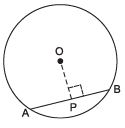⇒ AP =(1/2)AB
⇒ AP = (1/2)x 10 cm = 5 cm

Question 3. In the figure AOC is a diameter of the circle and arc AXB = (1/2) arc BYC. Find ∠BOC.
Solution:
∵ arc AXB = (1/2)arc BYC
∴ ∠AOB = (1/2) ∠BOC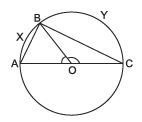Also ∠AOB + ∠BOC = 180°
⇒  (1/2)∠BOC + ∠BOC = 180°
⇒  (3/2) ∠BOC = 180°
⇒ ∠BOC =  (1/2) × 180° = 120°

Question 4. In the figure ∠ABC = 45°. Prove that OA ⊥ OC.
Solution:
Since the angle subtended at the centre by an arc is double the angle subtended by it at any other point on the remaining part of the circle.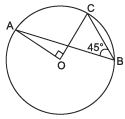∴ ∠ABC =(1/2)∠AOC
⇒ ∠AOC = 2 ∠ABC = 2 × 45° = 90°                  [∵ ∠ABC = 45°]
Thus, OA ⊥ OC.

Question 5. Look at the adjoining figure, in which O is the centre of the circle. If AB = 8 cm and OP = 3 cm, then find the radius of the circle.
Solution:
∵ OP ⊥ AB
∴ P is the mid-point of AB.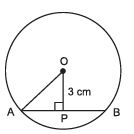⇒ AP = (1/2)AB = (1/2)x 8 cm = 4 cm
Now, in right ΔOPA, Radius, OA =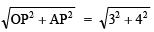= 5 cm

Question 6. In the adjoining figure, O is the centre of the circle. Find the length of AB.
Solution:
Since chord AB and chord CD subtend equal angles at the centre,
i.e. ∠ AOB = ∠ COD                  [Each = 60°]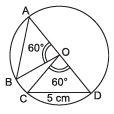∴ Chord AB = Chord CD
⇒ Chord AB = 5 cm                  [∵ Chord CD = 5 cm]
Thus, the required length of chord AB is 5 cm.

Question 7. In the adjoining figure, O is the centre of the circle and OP = OQ. If AP = 4 cm, then find the length of CD.
Solution:
∵ OP = OQ
∴ Chord AB and chord CD are equidistant from the centre.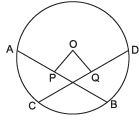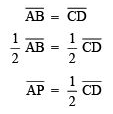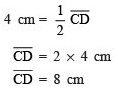Thus, the required length of CD is 8 cm.

Question 8. AB and CD are two parallel chords of a circle that are on opposite sides of the centre such that AB = 24 cm and CD = 10 cm and the distance between AB and CD is 17 cm. Find the radius of the circle.
Solution:
∵ Perpendicular from the centre to a chord bisects the chord.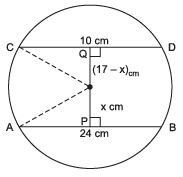∴ AP = (1/2)AB = (1/2)x 24 cm
= 12 cm
Similarly, CQ = (1/2)CD =(1/2)x 10 cm = 5 cm
Let OP = x cm
⇒ OQ = (17 - x) cm
Now, in right ΔAPO, x+ 122 = OA2                  ...(1)
Again, in right ΔCOQ, OC= CQ2 + (17 - x)2
= 5+ 172 + x2 - 34x
= 25 + 289 + x2 - 34x = 314 + x2 - 34x                  ...(2)
From (1) and (2),
we have x2 + 314 - 34x = x2 + 122
⇒ x2 - x2 - 34x = 144 - 314
⇒ - 34x = -170
⇒ x= (-170/34)  = 5

Now from (1), we have OA= 52 + 122 = 25 + 144
⇒ OA= 169
⇒ OA = 13 cm
Thus, the required radius of the circle = 13 cm.

Question 9. If O is the centre of the circle, then find the value of x.
Solution:
∵ AOB is a diameter.
∴ ∠ AOC + ∠ COB = 180°                  [Linear pairs]
⇒ 130° + ∠ COB = 180°
⇒ ∠ COB = 180° - 130° = 50°
Now, the arc CB is subtending ∠COB at the centre and ∠CDB at the remaining part.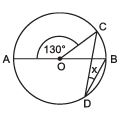∴ ∠ CDB = (1/2)∠COB
⇒ ∠ CDB = (1/2)x 50° = 25°
Thus, the measure of x = 25°

Question 10. The radius of a circle is 17 cm. A chord of length 30 cm is drawn. Find the distance of the chord from the centre.
Solution:
Length of chord AB = 30 cm.
Since, OP ⊥ AB
∴ P is the mid-point of AB.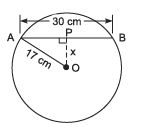AP = (1/2)AB =(1/2) x 30 cm = 15 cm
Now, in right ΔAPO, AO2 = AP2 + OP2
⇒ 172 = 15+ OP2
∴ OP2 = 172 - 152 = (17 - 15)(17 + 15)
= 2 x 32 = 64
⇒ OP = 64 = 8 cm
∴ The distance of the chord AB from the centre O is 8 cm.

Question 11. Find the length of a chord which is at a distance of 4 cm from the centre of a circle of radius 5 cm.
Solution:
∵ The perpendicular distance, OP = 4 cm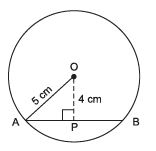∴ In right ΔAPO, AO2 = AP2 + OP2
⇒ 52 = AP+ 42
⇒ AP2 = 5- 4= (5 - 4)(5 + 4)
= 1 x 9 = 9
⇒ AP = √9= 3 cm
Since the perpendicular from the centre to a chord of a circle divides the chord into two equal parts.
∴ AP =  (1/2) AB
⇒ AB = 2AP
⇒ AB = 2 x 3 cm = 6 cm
Thus, the required length of chord AB is 6 cm.

Offer running on EduRev: Apply code STAYHOME200 to get INR 200 off on our premium plan EduRev Infinity!

## Mathematics (Maths) Class 9

47 videos|324 docs|82 tests

,

,

,

,

,

,

,

,

,

,

,

,

,

,

,

,

,

,

,

,

,

;